MAHARASHTRA

XII (12) HSC

XI (11) FYJC
X (10) SSC

### UNIT EXERCISE 1, RELATIONS AND FUNCTIONS, 10TH MATHS NEW SYLLABUS, TAMIL NADU, TN,

Question 1 :
If the ordered pairs (x2 − 3x, y2 + 4y) and (-2,5) are equal, then find x and y.
Solution :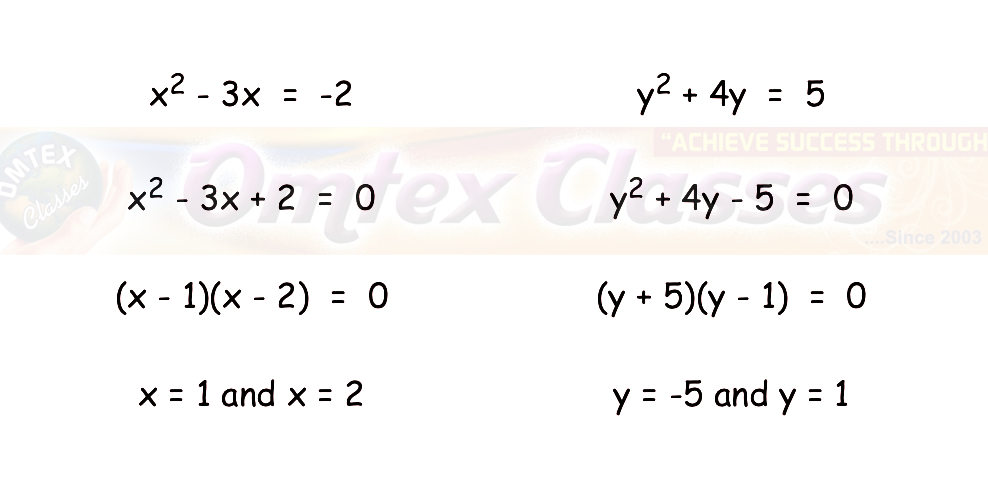Question 2 :
The Cartesian product A×A has 9 elements among which (–1, 0) and (0,1) are found. Find the set A and the remaining elements of A×A.

Solution :
So, in this question since A X A has 9 elements, so the number of elements in the set  A must obviously be 3.
Since the given elements of the cross product have -1, 0 and 1 as part of the entries, clearly these only must be the elements of the set A.
So A = {-1, 0, 1}
A X A
=  {(-1, -1),(-1, 0),(-1, 1),(0, -1),(0, 0),(0, 1),(1, -1),(1, 0),(1, 1)}

Question 3 :
Given that f(x)  =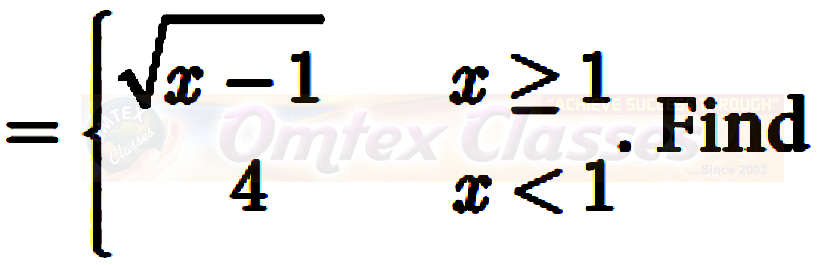(i) f (0) (ii) f (3) (iii) f (a+1) in terms of a.(Given that a   0)
Solution :
(i)  f(0)
Instead of x, we have 0, it is less than 1
f(0)  = 4
(ii)  f(3)
Instead of x, we have 3, it is greater than 1
f(3)  = √(3 - 1)
f(3)  = √2
(iii) f (a+1)
Instead of x, we have a+1, it is greater than 1
f(a + 1)  = √(a + 1 - 1)
f(a + 1)  = √a
Question 4 :
Let A= {9, 10, 11, 12, 13, 14, 15, 16, 17} and let f : A-> N be defined by f (n) = the highest prime factor of n A. Write f as a set of ordered pairs and find the range of f.
Solution :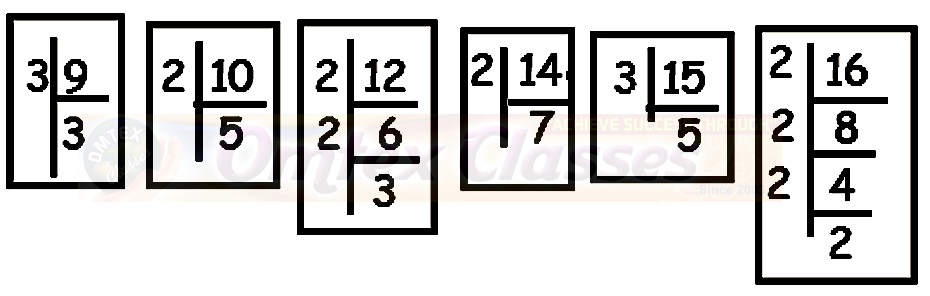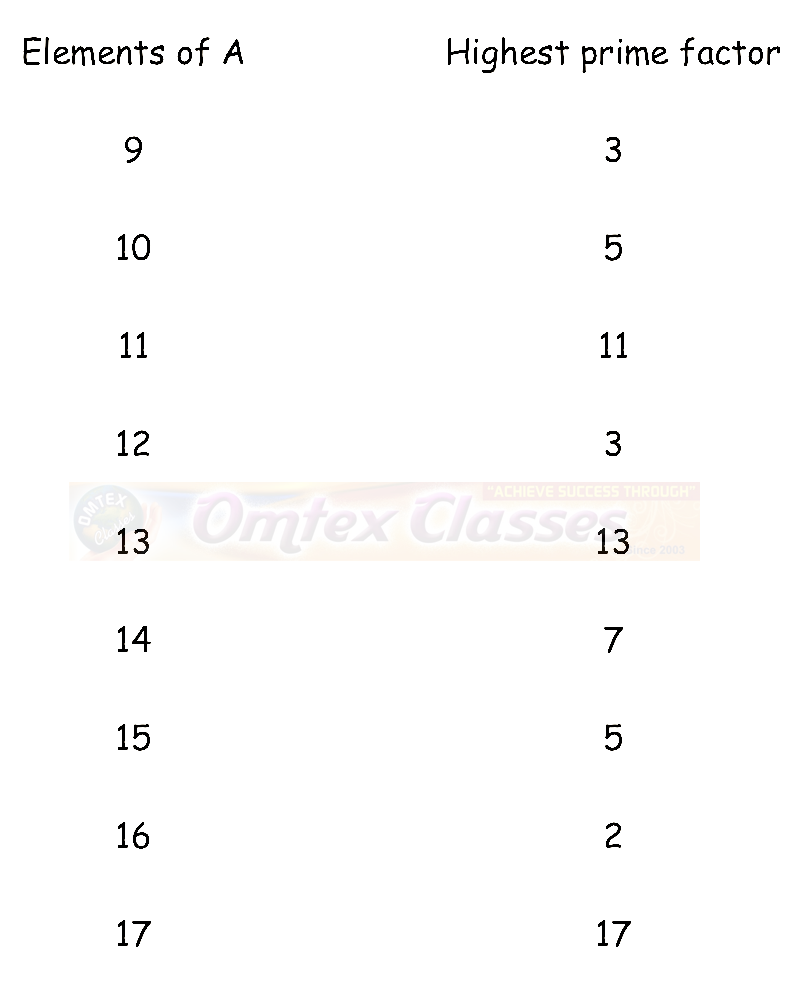Set ordered pairs :
=  {(9,3) (10, 5)(11, 11)(12, 3)(13, 13) (14, 7) (15, 5) (16,2) (17, 17)}
Range  = {2, 3, 5, 7,  11, 13, 17}

Question 5 :
Find the domain of the function f(x) =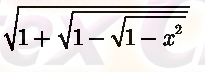Solution :
Let t = √(1 - √(1 - x2))
f(x)  = √(1 - t)
1 - t ≥ 0
t ≤ 1
By applying the value of t, we get
√(1 - √(1 - x2)) ≤ 1
1 - √(1 - x2)) ≤ 1
Subtracting 1 through out the equation,
- √(1 - x2)) ≤ 0
√(1 - x2)) ≥0
Taking squares on both sides,
(1 - x2)  ≥ 0
- x2 ≥ -1
x ≤ 1
Hence the domain is x ∈ [-1, 1]

Question 6 :
If f (x) = x2 , g(x) = 3x and h(x) = x −2 , Prove that (f g) h = f (g h) .
Solution :
L.H.S :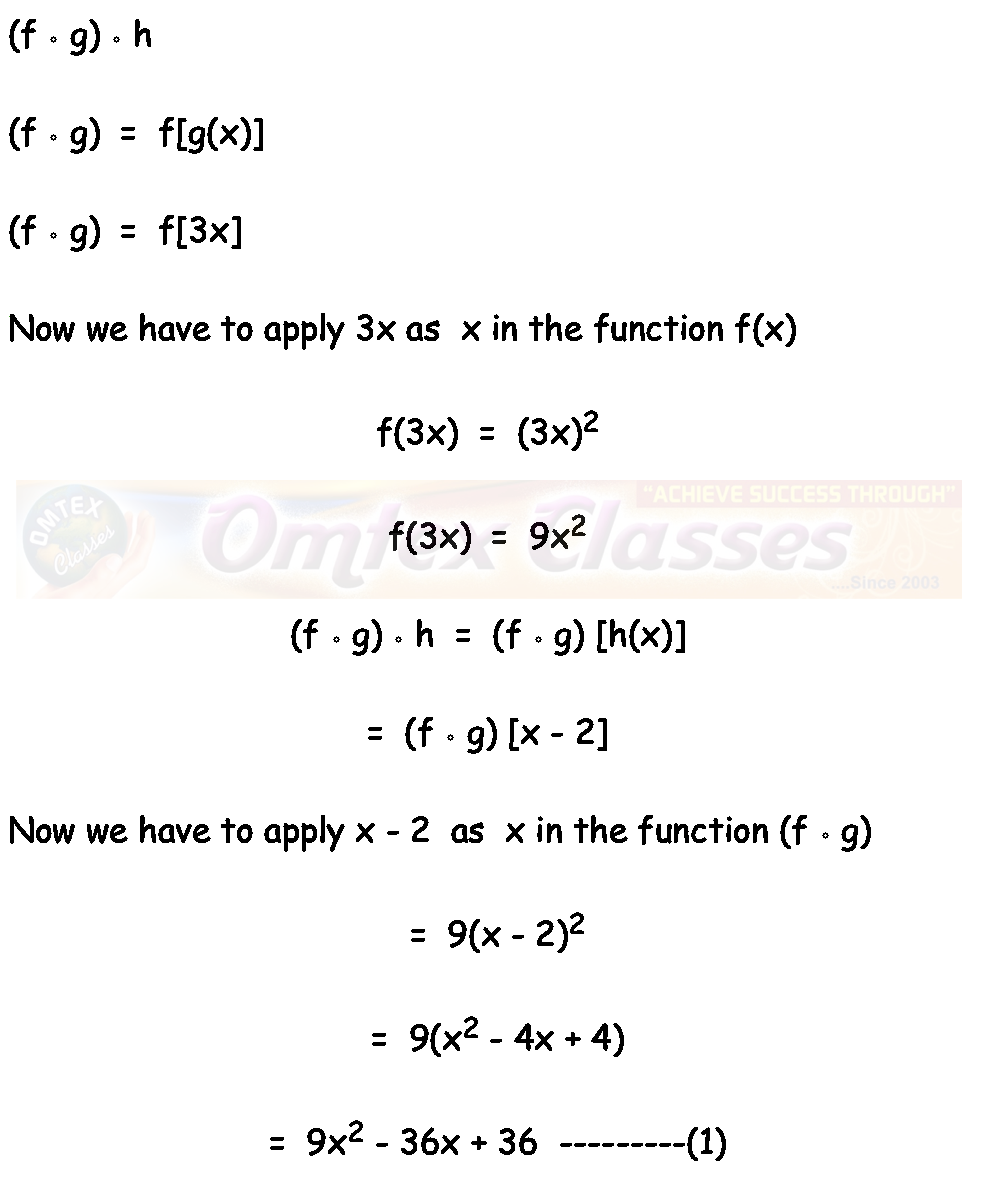R.H.S :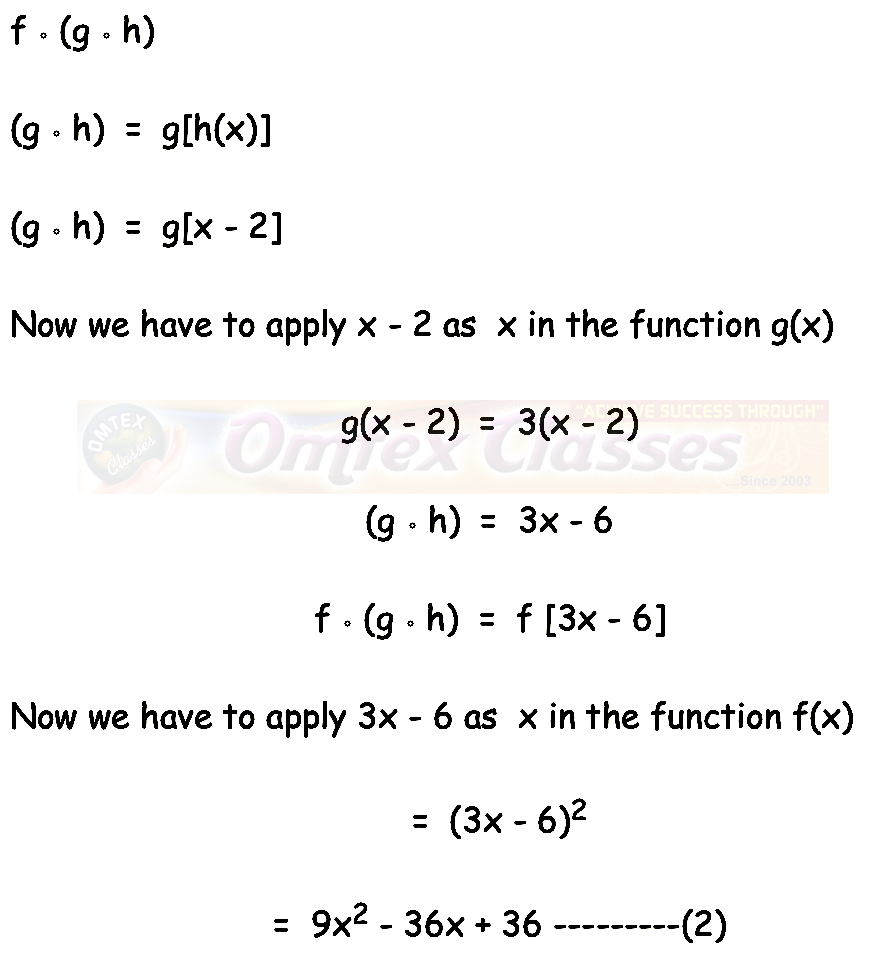Hence proved.

Question 7 :
Let A = {1, 2} and B = {1, 2, 3, 4} , C = {5, 6} and D = {5, 6 ,7, 8} . Verify whether A × C is a subset of B × D?
Solution :
A = {1, 2} and C = {5, 6}
A x C  = {(1, 5) (1, 6) (2, 5) (2, 6)}  ----(1)
B = {1, 2, 3, 4} and D = {5, 6 ,7, 8}
B x D  = { (1, 5) (1, 6) (1, 7) (1, 8) (2, 5) (2, 6) (2, 7) (2, 8) (3, 5) (3, 6) (3, 7) (3, 8) (4, 5) (4, 6) (4, 7) (4, 8) }
Hence A x C is the subset of B x D.

Question 8 :
If f(x)  = (x - 1)/(x + 1), x ≠ 1 show that f(f(x))  = -1/x, provided x ≠ 0
Solution :
f(x)  = (x - 1)/(x + 1) (Given)
f(f(x))  =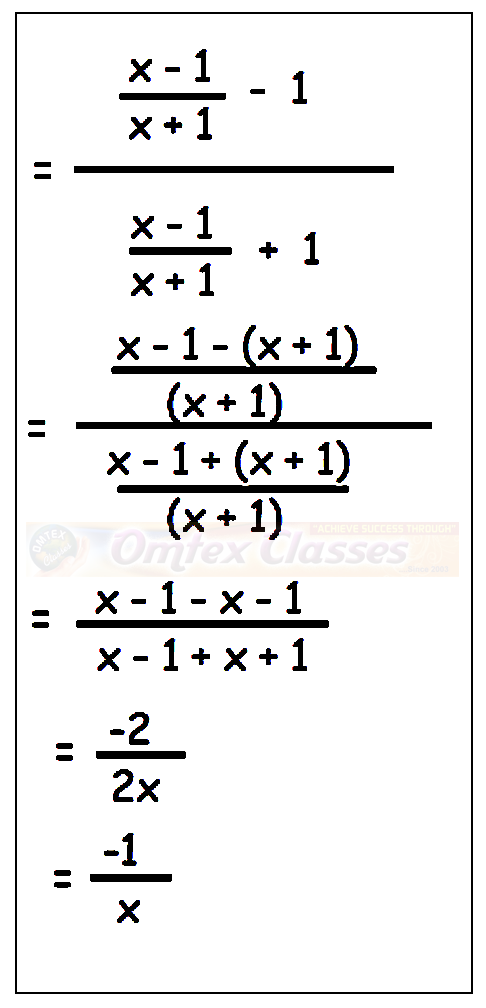Hence proved.

Question 9 :
The functions f and g are defined by f (x) = 6x + 8; g (x)  = (x - 2)/3
(i) Calculate the value of gg (1/2)
(ii) Write an expression for gf (x) in its simplest form.
Solution :
g (x)  = (x - 2)/3
g(1/2)  = ((1/2) - 2)/3
=  (-3/2)/3
g(1/2)   = -1/2
gg(1/2)  = ((-1/2) - 2)/3
=  (-5/2)/3
gg(1/2)  = -5/6

(ii) Write an expression for gf (x) in its simplest form.
gf (x)  = g[6x +8]
Now we have to apply 6x + 8 instead of x in g(x).
=  [(6x + 8) - 2]/3
=  (6x + 6)/3
=  2x + 2
=  2 (x + 1)

Question 10 :
Write the domain of the following real functions
(i) f (x)  = (2x + 1)/(x - 9)
Solution :
To find the domain, let us equate the denominator to 0
x - 9 = 0
x  = 9
The function is defined for all real values of x except 9.
Hence the required domain is R - {9}.

(ii)  p(x) =  -5/(4x2 + 1)
Solution :
In the denominator, we have x2. For all real values of x, we get positive values. Hence the required domain is R.

(iii)  g(x) =  √(x - 2)
Solution :
Since the given function is in radical sign, we should not get negative answer. For that we have to apply the values grater than 2.
Hence the required domain for he given function is [2, ∞)

(iv) h(x)  = x + 6
Solution :
For all real values of x, we get defined values of h(x). Hence the domain is R.

## PDF FILE TO YOUR EMAIL IMMEDIATELY PURCHASE NOTES & PAPER SOLUTION. @ Rs. 50/- each (GST extra)

SUBJECTS

HINDI ENTIRE PAPER SOLUTION

MARATHI PAPER SOLUTION
SSC MATHS I PAPER SOLUTION
SSC MATHS II PAPER SOLUTION
SSC SCIENCE I PAPER SOLUTION
SSC SCIENCE II PAPER SOLUTION
SSC ENGLISH PAPER SOLUTION
SSC & HSC ENGLISH WRITING SKILL
HSC ACCOUNTS NOTES
HSC OCM NOTES
HSC ECONOMICS NOTES
HSC SECRETARIAL PRACTICE NOTES

2019 Board Paper Solution

HSC ENGLISH SET A 2019 21st February, 2019

HSC ENGLISH SET B 2019 21st February, 2019

HSC ENGLISH SET C 2019 21st February, 2019

HSC ENGLISH SET D 2019 21st February, 2019

SECRETARIAL PRACTICE (S.P) 2019 25th February, 2019

HSC XII PHYSICS 2019 25th February, 2019

CHEMISTRY XII HSC SOLUTION 27th, February, 2019

OCM PAPER SOLUTION 2019 27th, February, 2019

HSC MATHS PAPER SOLUTION COMMERCE, 2nd March, 2019

HSC MATHS PAPER SOLUTION SCIENCE 2nd, March, 2019

SSC ENGLISH STD 10 5TH MARCH, 2019.

HSC XII ACCOUNTS 2019 6th March, 2019

HSC XII BIOLOGY 2019 6TH March, 2019

HSC XII ECONOMICS 9Th March 2019

SSC Maths I March 2019 Solution 10th Standard11th, March, 2019

SSC MATHS II MARCH 2019 SOLUTION 10TH STD.13th March, 2019

SSC SCIENCE I MARCH 2019 SOLUTION 10TH STD. 15th March, 2019.

SSC SCIENCE II MARCH 2019 SOLUTION 10TH STD. 18th March, 2019.

SSC SOCIAL SCIENCE I MARCH 2019 SOLUTION20th March, 2019

SSC SOCIAL SCIENCE II MARCH 2019 SOLUTION, 22nd March, 2019

XII CBSE - BOARD - MARCH - 2019 ENGLISH - QP + SOLUTIONS, 2nd March, 2019

HSC Maharashtra Board Papers 2020

(Std 12th English Medium)

HSC ECONOMICS MARCH 2020

HSC OCM MARCH 2020

HSC ACCOUNTS MARCH 2020

HSC S.P. MARCH 2020

HSC ENGLISH MARCH 2020

HSC HINDI MARCH 2020

HSC MARATHI MARCH 2020

HSC MATHS MARCH 2020

SSC Maharashtra Board Papers 2020

(Std 10th English Medium)

English MARCH 2020

HindI MARCH 2020

Hindi (Composite) MARCH 2020

Marathi MARCH 2020

Mathematics (Paper 1) MARCH 2020

Mathematics (Paper 2) MARCH 2020

Sanskrit MARCH 2020

Important-formula

THANKS